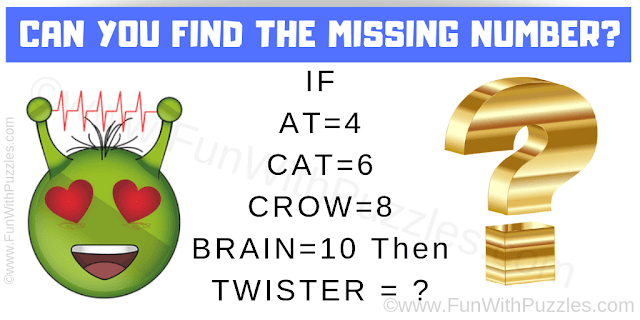This is very interesting maths logical questions for high school students. In this maths logic puzzle, you are shown some equations in which some words equate to the numbers. Your challenge is to find the logical pattern in these given equations and then find the value of the missing number which will replace the question mark.Can you solve this Maths Logical Question?

The answer to this "Maths Logical Question", can be viewed by clicking on the answer button.